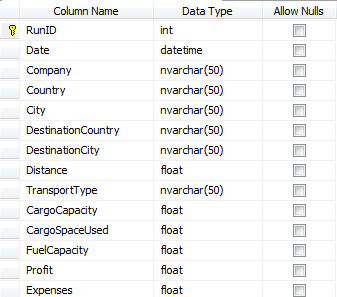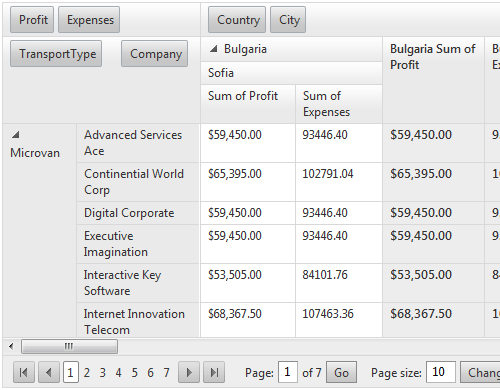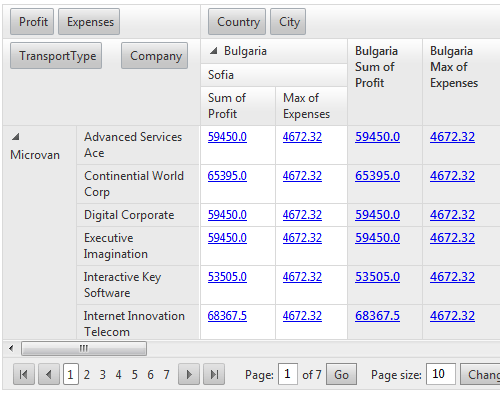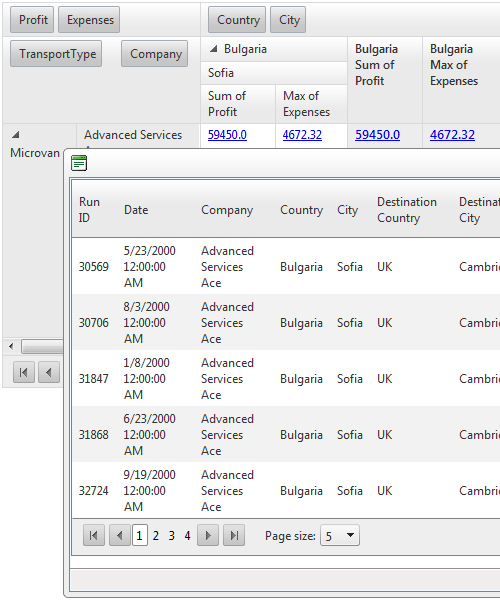This blog post is the first of two blog posts that will show you how to implement a drill-down functionality into RadPivotGrid and then how to optimize it for best performance.

Let’s get straight to the question:

## What is drill down?

The drill-down capability enables you to retrieve the complete list of records that is used to calculate a particular summary in a PivotGrid’s data cells. By implementing drill down in Telerik’s ASP.NET PivotGrid control you can move from summary information presented in each data cell to detailed data which is used for the calculation of this cell’s value.

## Why drill down?

Since the time when we released the first version of RadPivotGrid, we have been receiving requests for implementing a functionality which will give you the power to obtain the underline data related to the calculated value in each data cell. The good news is that with the Q1 2013 SP1 release of our ASP.NET controls, the RadPivotGrid is powerful enough and you can achieve such functionality by yourself. Here’s how.

## Initial setup

Let’s create a simple RadPivotGrid bound to a database table via Advanced Data Binding:

`<``telerik:RadPivotGrid` `Width``=``"800px"` `runat``=``"server"` `ID``=``"RadPivotGrid1"`
`             ``AllowPaging``=``"true"` `AllowFiltering``=``"false"` `ShowFilterHeaderZone``=``"false"` `OnNeedDataSource``=``"RadPivotGrid1_NeedDataSource"``>`
`            ``<``PagerStyle` `Mode``=``"NextPrevNumericAndAdvanced"` `AlwaysVisible``=``"true"``></``PagerStyle``>`
`            ``<``Fields``>`
`                ``<``telerik:PivotGridRowField` `DataField``=``"TransportType"``>`
`                ``</``telerik:PivotGridRowField``>`
`                ``<``telerik:PivotGridRowField` `DataField``=``"Company"``>`
`                ``</``telerik:PivotGridRowField``>`
`                ``<``telerik:PivotGridColumnField` `DataField``=``"Country"``>`
`                ``</``telerik:PivotGridColumnField``>`
`                ``<``telerik:PivotGridColumnField` `DataField``=``"City"``>`
`                ``</``telerik:PivotGridColumnField``>`
`                ``<``telerik:PivotGridAggregateField` `DataField``=``"Profit"` `Aggregate``=``"Sum"` `>`
`                ``</``telerik:PivotGridAggregateField``>`
`                ``<``telerik:PivotGridAggregateField` `DataField``=``"Expenses"` `Aggregate``=``"Max"``>`
`                ``</``telerik:PivotGridAggregateField``>`
`            ``</``Fields``>`
`            ``<``ClientSettings``>`
`                ``<``Scrolling` `AllowVerticalScroll``=``"true"``></``Scrolling``>`
`            ``</``ClientSettings``>`
`        ``</``telerik:RadPivotGrid``>`

`  ``protected void RadPivotGrid1_NeedDataSource(object sender, PivotGridNeedDataSourceEventArgs e)`
`    ``{`
`        ``(sender as RadPivotGrid).DataSource = GetDataTable("SELECT * FROM Transportation");`
`    ``}`

`    ``public DataTable GetDataTable(string query)`
`    ``{`
`        ``String ConnString = ConfigurationManager.ConnectionStrings["TelerikConnectionString"].ConnectionString;`
`        ``SqlConnection conn = new SqlConnection(ConnString);`
`        ``SqlDataAdapter adapter = new SqlDataAdapter();`
`        ``adapter.SelectCommand = new SqlCommand(query, conn);`

`        ``DataTable myDataTable = new DataTable();`

`        ``conn.Open();`
`        ``try`
`        ``{`
`            ``adapter.Fill(myDataTable);`
`        ``}`
`        ``finally`
`        ``{`
`            ``conn.Close();`
`        ``}`

`        ``return myDataTable;`
`    ``}`

## The database and how the PivotGrid will look

The Transportation table has the following columns:Here is how the resulting PivotGrid looks:## Interaction with the data cells

Now we need some interaction with the data cells in order to fire a post back and get the corresponding data. One possible option here is to use LinkButtons instead of plain text into the cells. To add LinkButtons into each cell we have two options - 1. Use PivotGrid templates (but this is too easy :) ) and 2. Insert controls into the data cells with code (which I will show you below).

`protected override void OnInit(EventArgs e)`
`    ``{`
`        ``base.OnInit(e);`

`        ``RadPivotGrid1.ItemCommand += RadPivotGrid1_ItemCommand;`
`        ``RadPivotGrid1.CellCreated += RadPivotGrid1_CellCreated;`
`        ``RadPivotGrid1.CellDataBound += RadPivotGrid1_CellDataBound;`
`    ``}`

`void RadPivotGrid1_CellCreated(object sender, PivotGridCellCreatedEventArgs e)`
`    ``{`
`        ``PivotGridDataCell cell = e.Cell as PivotGridDataCell;`
`        ``if (cell != null)`
`        ``{`
`            ``cell.Controls.Add(new LinkButton() { ID = "LinkButton1" });`
`        ``}`
`    ``}`

Then in RadPivotGrid1_CellDataBound we need to find the LinkButton and assign the data cell DataItem as a text.

`void RadPivotGrid1_CellDataBound(object sender, PivotGridCellDataBoundEventArgs e)`
`    ``{`
`        ``PivotGridDataCell cell = e.Cell as PivotGridDataCell;`
`        ``if (cell != null)`
`        ``{`
`            ``LinkButton button = cell.FindControl("LinkButton1") as LinkButton;`
`            ``if (cell.DataItem != null)`
`            ``{`
`                ``button.Text = cell.DataItem.ToString();`
`            ``}`
`            ``else`
`            ``{`
`                ``button.Visible = false;`
`            ``}`
`        ``}`
`    ``}`

Here is the result:## Visualizing the detailed data

Now, how to visualize the detailed data for each data cell when the LinkButton is clicked? The answer is simple: we need to use another major control which displays tabular data – Telerik’s ASP.NET Grid. Also, in order for the application to be more intuitive we can place the RadGrid into an instance of Telerik’s Popup Dialog for ASP.NET, RadWindow, and to show a window when someone clicks on the LinkButtons:

`<``telerik:RadWindow` `ID``=``"RadWindow1"` `runat``=``"server"` `MinWidth``=``"900px"` `MinHeight``=``"450px"``>`
`            ``<``ContentTemplate``>`
`                   ``<``telerik:RadAjaxLoadingPanel` `runat``=``"server"` `ID``=``"RadAjaxLoadingPanel2"` `Skin``=``"Default"``></``telerik:RadAjaxLoadingPanel``>`
`                ``<``telerik:RadAjaxPanel` `runat``=``"server"` `LoadingPanelID``=``"RadAjaxLoadingPanel2"``>`
`                    ``<``telerik:RadGrid` `runat``=``"server"` `ID``=``"RadGrid1"` `AllowSorting``=``"true"` `PageSize``=``"5"`
`                        ``OnNeedDataSource``=``"RadGrid1_NeedDataSource"` `AllowPaging``=``"true"``>`
`                    ``</``telerik:RadGrid``>`
`                ``</``telerik:RadAjaxPanel``>`
`            ``</``ContentTemplate``>`
`        ``</``telerik:RadWindow``>`

Now how to bind the RadGrid and how to show the window? It is very easy - the RadGrid can be bound via Advanced Data Binding with NeedDataSourceEvent:

`protected void RadGrid1_NeedDataSource(object sender, GridNeedDataSourceEventArgs e)`
`    ``{`
`        ``RadGrid1.DataSource = GridDataSource;`
`    ``}`

Where the GridDataSource property has the following declaration:

`public DataTable GridDataSource`
`    ``{`
`        ``get`
`        ``{`
`            ``return Session["GridDataSource"] as DataTable;`
`        ``}`
`        ``set`
`        ``{`
`            ``Session["GridDataSource"] = value;`
`        ``}`
`    ``}`

Why do we need to keep the grid data source into the Session? Because if we bind the RadGrid when a LinkButton is clicked, on every post back raised from the RadGrid (for example paging) the RadGrid data will be empty because the grid is recreated from the ViewState.

So now we have RadGrid and its data source, but how to show the RadWindow and the RadGrid into it? One of the possible options here is to handle:

`void RadPivotGrid1_CellDataBound(object sender, PivotGridCellDataBoundEventArgs e)`
`    ``{`
`        ``PivotGridDataCell cell = e.Cell as PivotGridDataCell;`
`        ``if (cell != null)`
`        ``{`
`            ``…`
`            ``button.CommandName = "OpenDetailsWindow";`
`…`
`        ``}`
`    ``}`

If the e.CommandName is “OpenDetailsWindow”, then we need to add DataTable with corresponding data to GridDataSource, call RadGrid.Rebind() method and set a RadWindow1.VisibleOnPageLoad property to false:

`void RadPivotGrid1_ItemCommand(object sender, PivotGridCommandEventArgs e)`
`    ``{`
`        ``if (e.CommandName == "OpenDetailsWindow")`
`        ``{`
`            `
`     ``GridDataSource = // Get data`
`            ``RadWindow1.VisibleOnPageLoad = true;`
`            ``RadGrid1.Rebind();`
`        ``}`
`        ``else`
`        ``{`
`            ``RadWindow1.VisibleOnPageLoad = false;`
`        ``}`
`    ``}`

## Get the corresponding data

So far so good, we have RadPivotGrid, LinkButtons in every data cell, RadGrid bound to a DataTable and shown when a LinkButton is clicked. But what am I missing…? O yeah, how to get the corresponding to each cell data? This is the tricky part because we need to perform additional call to the database and get this data which is related to the data cell’s calculated value. One possible approach could be to get all corresponding to the data cell ParentRowIndexes and ParentColumnIndexes and build the query based on them. The indexes can be passed as ComamndArgument of the LinkButton:

`void RadPivotGrid1_CellDataBound(object sender, PivotGridCellDataBoundEventArgs e)`
`    ``{`
`        ``PivotGridDataCell cell = e.Cell as PivotGridDataCell;`
`        ``if (cell != null)`
`        ``{`
`            ``LinkButton button = cell.FindControl("LinkButton1") as LinkButton;`
`            ``button.CommandName = "OpenDetailsWindow";`
`            ``button.CommandArgument = GetCommandArguments(cell);`
`            ``if (cell.DataItem != null)`
`            ``{`
`                ``button.Text =  cell.DataItem.ToString();`
`            ``}`
`            ``else`
`            ``{`
`                ``button.Visible = false;`
`            ``}`
`        ``}`
`    ``}`

`    ``List<``PivotGridField``> rowFields;`
`    ``List<``PivotGridField``> columnFields;`
`    ``List<``PivotGridField``> aggregateFields;`

`    ``protected override void OnLoad(EventArgs e)`
`    ``{`
`        ``base.OnLoad(e);`

`        ``rowFields = RadPivotGrid1.Fields.Where(f =>`
`        ``f is PivotGridRowField && f.IsHidden == false).OrderBy(f => f.ZoneIndex).ToList();`
`        ``columnFields = RadPivotGrid1.Fields.Where(f =>`
`             ``f is PivotGridColumnField && f.IsHidden == false).OrderBy(f => f.ZoneIndex).ToList();`
`        ``aggregateFields = RadPivotGrid1.Fields.Where(f =>`
`             ``f is PivotGridAggregateField && f.IsHidden == false).OrderBy(f => f.ZoneIndex).ToList();`
`    ``}`

Where the GetCommandArguments looks like:

`public string GetCommandArguments(PivotGridDataCell cell)`
`    ``{`
`        ``object[] rowIndexes = cell.ParentRowIndexes;`
`        ``object[] columnIndexes = cell.ParentColumnIndexes;`
`        ``string fieldName = cell.Field.DataField;`

`        ``int rowIndexesCount = rowIndexes.Count();`
`        ``int columnIndexesCount = columnIndexes.Count();`

`        ``if (cell.CellType == PivotGridDataCellType.RowAndColumnGrandTotal)`
`        ``{`
`            ``return string.Empty;`
`        ``}`

`        ``AdjustRowAndColumnIndexes(cell, ref rowIndexesCount, ref columnIndexesCount);`

`        ``StringBuilder buider = new StringBuilder();`

`        ``for (int i = 0; i < ``rowIndexesCount``; i++)`
`        ``{`
`            ``string ``group` `= ``string``.Format("{0}~{1};", rowFields[i].DataField, rowIndexes[i].ToString());`
`            ``buider.Append(group);`
`        ``}`

`        ``for (int ``i` `= ``0``; i < columnIndexesCount; i++)`
`        ``{`
`            ``string ``group` `= ``string``.Format("{0}~{1};", columnFields[i].DataField, columnIndexes[i].ToString());`
`            ``buider.Append(group);`
`        ``}`

`        ``if (buider.Length > 1)`
`        ``{`
`            ``buider.Remove(buider.Length - 1, 1);`
`        ``}`

`        ``return buider.ToString();`
`    ``}`

Into it we build a complex command argument which has the following signature: “columnName~value;columnName~value”. In this way we can construct an SQL query which gets only the items related with cell value calculation. For example, if we click on the top left cell with value “59450.0” from our RadPivotGridthe argument will have the following signature: TransportType~Microvan;Company~Advanced Services Ace;Country~Bulgaria;City~Sofia

Into the GetCommandArguments we use AdjustRowAndColumnIndexes in order to adjust the column and row indexes count:

`private void AdjustRowAndColumnIndexes(PivotGridDataCell cell, ref int rowIndexesCount, ref int columnIndexesCount)`
`    ``{`
`        ``//if aggregates are more than one additional cells are rendered, so we need to exclude their values from the query`
`        ``if (aggregateFields.Count > 1)`
`        ``{`
`            ``if (RadPivotGrid1.AggregatesPosition == PivotGridAxis.Columns)`
`            ``{`
`                ``if (cell.CellType == PivotGridDataCellType.DataCell ||`
`                    ``cell.CellType == PivotGridDataCellType.RowTotalDataCell ||`
`                    ``cell.CellType == PivotGridDataCellType.RowAndColumnTotal ||`
`                    ``cell.CellType == PivotGridDataCellType.RowGrandTotalDataCell ||`
`                    ``cell.CellType == PivotGridDataCellType.ColumnGrandTotalRowTotal)`
`                ``{`
`                    ``columnIndexesCount--;`
`                ``}`
`            ``}`
`            ``else`
`            ``{`
`                ``if (cell.CellType != PivotGridDataCellType.RowTotalDataCell &&`
`                    ``cell.CellType != PivotGridDataCellType.ColumnGrandTotalRowTotal)`
`                ``{`
`                    ``rowIndexesCount--;`
`                ``}`
`            ``}`
`        ``}`

`        ``// if row total or grand total cell is hit we need to escape its values from query`
`        ``if (cell.CellType == PivotGridDataCellType.RowTotalDataCell ||`
`            ``cell.CellType == PivotGridDataCellType.ColumnGrandTotalRowTotal ||`
`            ``cell.CellType == PivotGridDataCellType.RowGrandTotalDataCell)`
`        ``{`
`            ``rowIndexesCount--;`
`        ``}`

`        ``// if column total or grand total cell is hit we need to escape its values from query`
`        ``if (cell.CellType == PivotGridDataCellType.ColumnTotalDataCell ||`
`            ``cell.CellType == PivotGridDataCellType.ColumnGrandTotalDataCell ||`
`            ``cell.CellType == PivotGridDataCellType.RowGrandTotalColumnTotal ||`
`            ``cell.CellType == PivotGridDataCellType.RowAndColumnTotal ||`
`            ``cell.CellType == PivotGridDataCellType.ColumnGrandTotalRowTotal)`
`        ``{`
`            ``columnIndexesCount--;`
`        ``}`
`    ``}`

We need this because the Row and Column parent indexes contain the values for the total and grand total header cells, but they do not need to be included into the “where” clause of the SQL query. They are generated from the PivotGrid engine and we do not need to use them in the drill down functionality. Also, if there is more than one aggregate field, we need to escape their corresponding values from the query , that’s why we need to get the code in if (aggregateFields.Count > 1) condition.

## Convert the passed arguments back

Now when we have the command argument, the only thing that remains is to parse it back into the RadPivotGrid1_ItemCommand event handler and to construct the SQL query:

`void RadPivotGrid1_ItemCommand(object sender, PivotGridCommandEventArgs e)`
`  ``{`
`      ``if (e.CommandName == "OpenDetailsWindow")`
`      ``{`
`          ``string[] groups = e.CommandArgument.ToString().Split(new char[] { '|' }, StringSplitOptions.RemoveEmptyEntries);`
`          ``StringBuilder wheres = new StringBuilder();`

`          ``if (groups.Count() > 0)`
`          ``{`
`              ``string[] elements = groups.Split(new char[] { ';' }, StringSplitOptions.RemoveEmptyEntries);`
`              ``foreach (var element in elements)`
`              ``{`
`                  ``var pairs = element.Split(new char[] { '~' }, StringSplitOptions.RemoveEmptyEntries);`
`                  ``wheres.Append(string.Format("{0} = '{1}' AND ", pairs, pairs));`
`              ``}`

`              ``wheres.Remove(wheres.Length - 5, 5);`

`              ``GridDataSource = GetDataTable(string.Format("SELECT * FROM Transportation WHERE {0}", wheres.ToString()));`
`          ``}`
`          ``//if the rowcolumngrandototal cell is clicked`
`          ``else`
`          ``{`
`              ``GridDataSource = GetDataTable("SELECT * FROM Transportation");`
`          ``}`

`          ``RadWindow1.VisibleOnPageLoad = true;`
`          ``RadGrid1.Rebind();`
`      ``}`
`      ``else`
`      ``{`
`          ``RadWindow1.VisibleOnPageLoad = false;`
`      ``}`
`  ``}`

With this our example is completed. When we run it and click on a LinkButton, the result will be:## Conclusion

I think that this post will help you start playing with the RadPivotGrid. Here I have shown you one of the possible approaches to build a drill-down functionality into the control, but you can try developing your own implementation. Also this is not the last post concerning the drill down support of RadPivotGrid. Tomorrow I will show you how to optimize the performance of this example, because having RadPivotGrid with 5,000 data cells is not a big deal, but having 5,000 data cells with a LinkButton into each cell is!

P.S. The example which demonstrated the described drill down functionality can be downloaded here